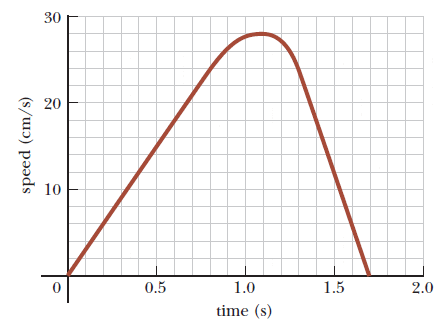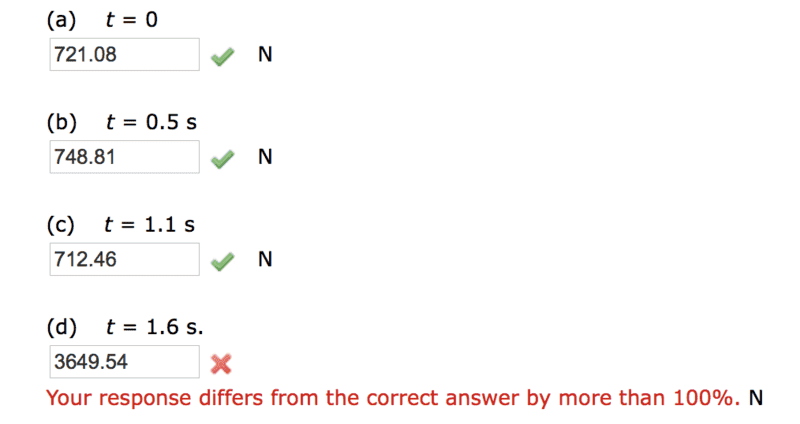# Difficult time with motion graphs & dynamics

## Homework Statement

The figure below shows the speed of a person's body as he does a chin-up. Assume the motion is vertical and the mass of the person's body is 72.7 kg. Determine the force exerted by the chin-up bar on his body at the following times.F = ma

## The Attempt at a Solution

I'm not quite sure how to solve that last part:

I solved for the acceleration at 1.6s which is -60m/s^2.

F = ma
-mg + T = m a
T = 72.7kg(60m/s^2) + (9.8m/s^2)(72.7kg)
T = 3649.54?? (this one's wrong)